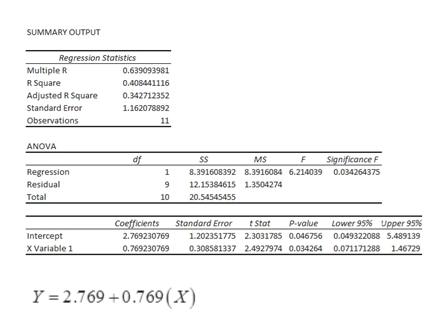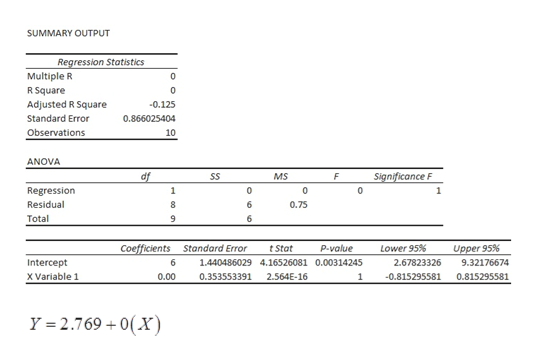# a. Using the pairs of values for all10​points, find the equation of the regression line.b. After removing the point with coordinatesleft parenthesis 1 comma 2 right parenthesis(1,2)​,use the pairs of values for the remaining99points and find the equation of the regression line.c. Compare the results from parts​ (a) and​ (b).    02468100246810xy    A scatterplot has a horizontal x-scale from 0 to 10 in increments of 1 and vertical y-scale from 0 to 10 in increments of 1. Ten points are plotted with nine points, (3, 5), (4, 5), (5, 5), (3, 6), (4, 6), (4, 6), (5, 6), (3, 7), (4, 7), and (5, 7), forming a square and the tenth point (1, 2) being below and to the left of the square.a. What is the equation of the regression line for all10​points? ModifyingAbove y with caretyequals=nothingplus+nothingx​ (Round to three decimal places as​ needed.)b. What is the equation of the regression line for the set of99​points? ModifyingAbove y with caretyequals=nothing​(Round to three decimal places as​ needed.)c. Choose the correct description of the results below.  A.The removal of the point has a significant impact on the regression line. B.There is no regression line for the second case because the data are in a pattern. C.The regression line​ changes, but the change is small. D.The regression line is very similar in both cases.

Question
2 views
 a. Using the pairs of values for all 10 ​points, find the equation of the regression line. b. After removing the point with coordinates left parenthesis 1 comma 2 right parenthesis(1,2)​, use the pairs of values for the remaining 99 points and find the equation of the regression line. c. Compare the results from parts​ (a) and​ (b). 02468100246810xy         A scatterplot has a horizontal x-scale from 0 to 10 in increments of 1 and vertical y-scale from 0 to 10 in increments of 1. Ten points are plotted with nine points, (3, 5), (4, 5), (5, 5), (3, 6), (4, 6), (4, 6), (5, 6), (3, 7), (4, 7), and (5, 7), forming a square and the tenth point (1, 2) being below and to the left of the square.
a. What is the equation of the regression line for all
10
​points?

ModifyingAbove y with caretyequals=nothingplus+nothingx
​ (Round to three decimal places as​ needed.)
b. What is the equation of the regression line for the set of
99
​points?

ModifyingAbove y with caretyequals=nothing
​(Round to three decimal places as​ needed.)
c. Choose the correct description of the results below.

A.
The removal of the point has a significant impact on the regression line.

B.
There is no regression line for the second case because the data are in a pattern.

C.
The regression line​ changes, but the change is small.

D.
The regression line is very similar in both cases.
check_circle

Step 1

Note:

There are 11 pairs of values in the data. Hence we are finding the regression line equation using 11 pairs of values.

Step 2

a.

Step-by step procedure to find the regression line using Excel software:

• Enter the give data into excel sheet.
• Select Data option in ribbon panel.
• Click on Data analysis, choose regression.
• Select the range of Y and X.
• Click ok.
• Output will be displayed.

The regression line using all 11 pairs of values is given below:help_outlineImage TranscriptioncloseSUMMARY OUTPUT Regression Statistics Multiple R 0.639093981 R Square Adjusted R Square 0.408441116 0.342712352 Standard Error 1.162078892 Observations 11 ANOVA Significance F df SS MS Regression 8.391608392 8.3916084 6.214039 0.034264375 Residual 9 12.15384615 1.3504274 10 Total 20.54545455 Standard Error 1.202351775 2.3031785 0.046756 0.049322088 5.489139 0.308581337 2.4927974 0.034264 0.071171288 146729 P-value Lower 95 % Upper 95% Coefficients 2.769230769 tStat Intercept Variable 1 0.769230769 Y 2.769 0.769(x fullscreen
Step 3

b.

The regression equation line after removing the point (1, 2) is obtained as follows.

Step-by step procedure to find the regression line using Excel software:

• Enter the give data into excel sheet.
• Select Data option in ribbon panel.
• Click on Data analysis, choose regression...help_outlineImage TranscriptioncloseSUMMARY OUTPUT Regression Statistics Multiple R 0 R Square 0 Adjusted R Square 0.125 0.866025404 Standard Error Observations 10 ANOVA Significance F df SS MS F Regression 1 0 0 1 Residual 8 6 0.75 Total 6 Upper 95 % Coefficients Standard Error t Stat Lower 95% p-value Intercept 6 1.440486029 4.16526081 0.00314245 2.67823326 9.32176674 X Variable 1 0.00 0.353553391 2.564E-16 1 0.815295581 0.815295581 Y =2.769 0(X) fullscreen

### Want to see the full answer?

See Solution

#### Want to see this answer and more?

Solutions are written by subject experts who are available 24/7. Questions are typically answered within 1 hour.*

See Solution
*Response times may vary by subject and question.
Tagged in

### Statistics